# How to Calculate a Daily Lease Liability Amortization Schedule in Excel

In this how-to guide, we’ll cover how to calculate a daily lease liability amortization schedule. This method shown is one of many ways of how to calculate a lease amortization schedule. The key attributes of this lease liability daily amortization schedule are:

• The most accurate way of calculating the lease amortization schedule (by far)
• It uses the XNPV function in Excel
• The calculation is done daily
• A more time-intensive setup but can handle any lease permutation

If you want to know more about present value calculations and the different Excel functions available, refer to How to Calculate the Present Value of Future Lease Payments

If you're looking for a quicker way to calculate your lease liability, refer to How to Calculate a Monthly Lease Liability Amortization Schedule in Excel. Between the two calculation methodologies, I'd recommend a daily lease liability amortization schedule. That's because it's not that much more of a time investment than the monthly NPV alternative. With the additional time investment, you have far the most accurate lease liability calculation, which allows you to handle any potential modification accounting.

If you would like the Excel calculations displayed in this article, reach out to [email protected].

## Lease Inputs

The lease agreement we’re going to calculate is based on the following details:

• Commencement date: January 1, 2021
• Lease end date: December 31, 2021
• Discount rate: 7%
• Fixed payment amount: \$10,000

With these inputs, we'll calculate the daily lease liability amortization schedule.

## Step 1 - Create the columns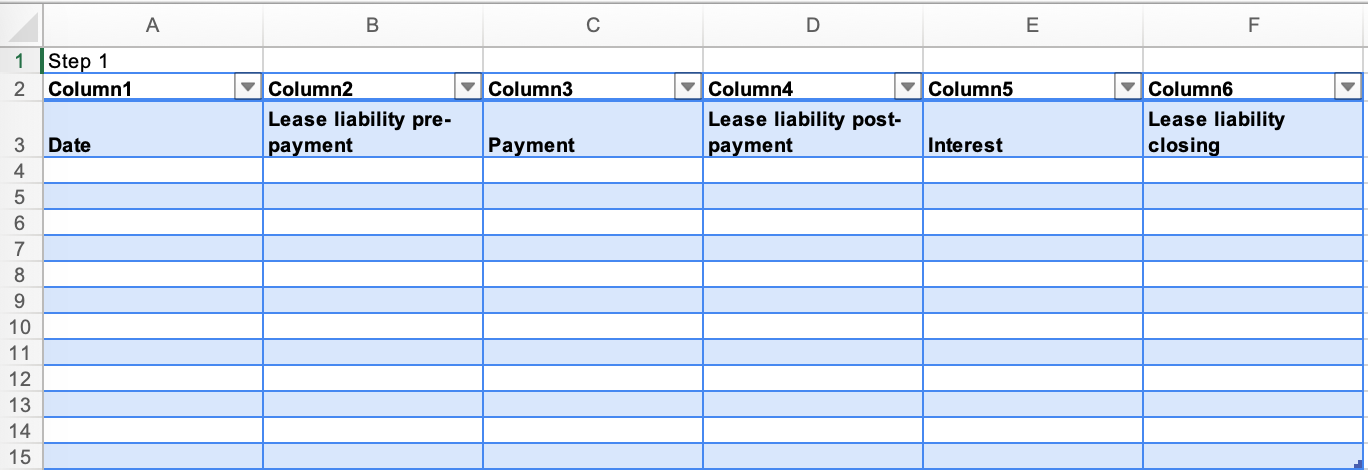• Create six columns within the Excel worksheet. The columns will be called Date, Lease liability pre-payment, Payment, Lease liability post-payment, Interest, and Lease liability closing.

## Step 2 - Input the applicable dates and payments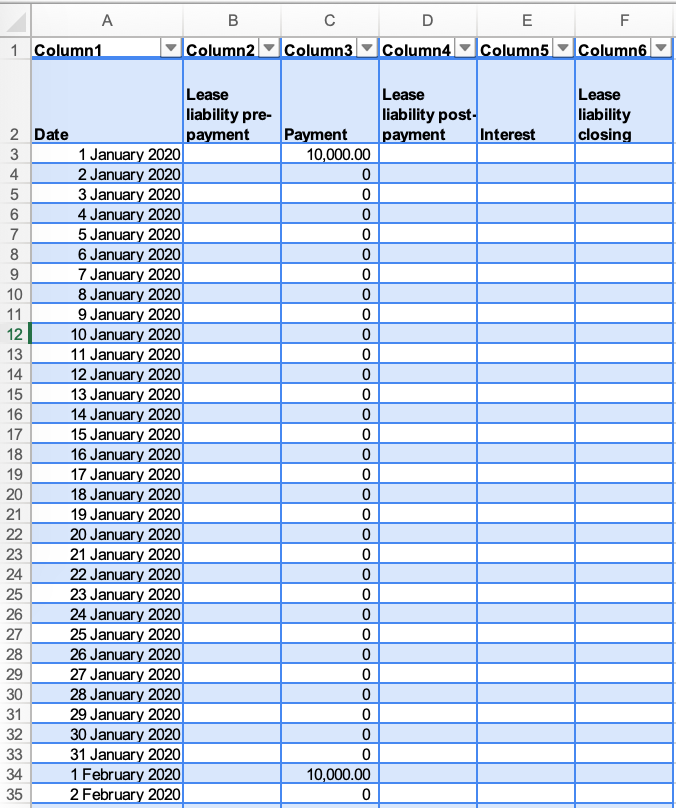• As this is a daily schedule, this will encompass all individual days of the lease. For example, a 12-month lease will have 365 rows. If the calculation falls on a leap year, the calculation will have 366 rows.
• These inputs are taken from the lease agreement. In this example, there are 12 payments occurring on the first of each month for an amount of \$10,000.

## Step 3 - Apply the XNPV function from Excel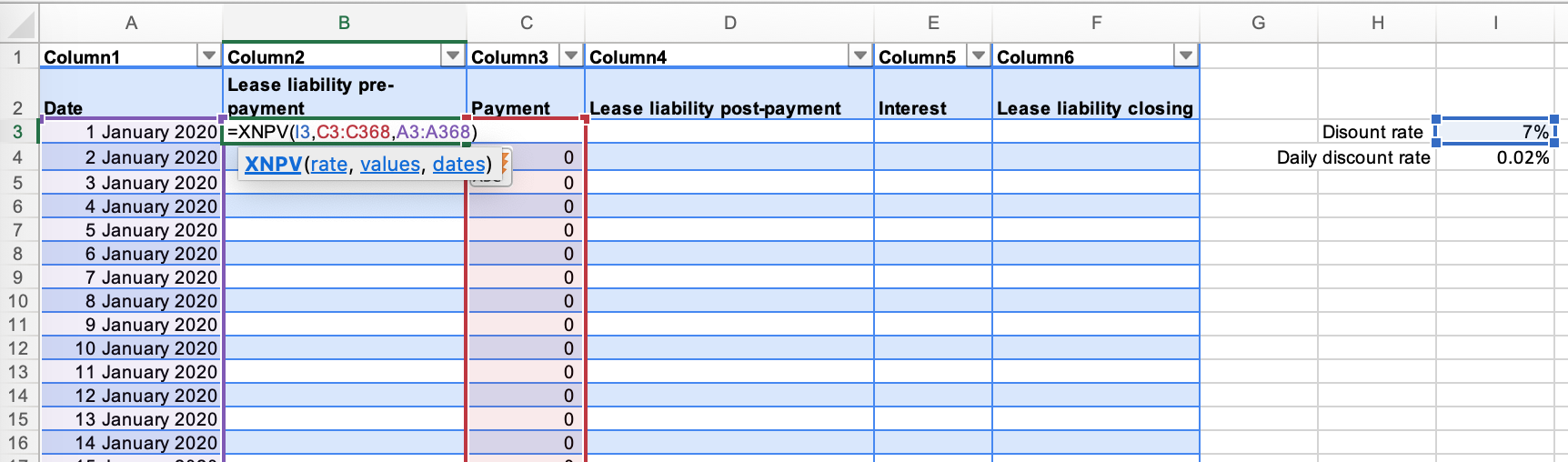• Input the XNPV function into Excel. The function captures the discount rate, the payment amounts, and the dates of the payments.
• The XNPV function is the most accurate present value formula due to taking into consideration the payment timing.

## Step 4 - Calculate the lease liability post-payment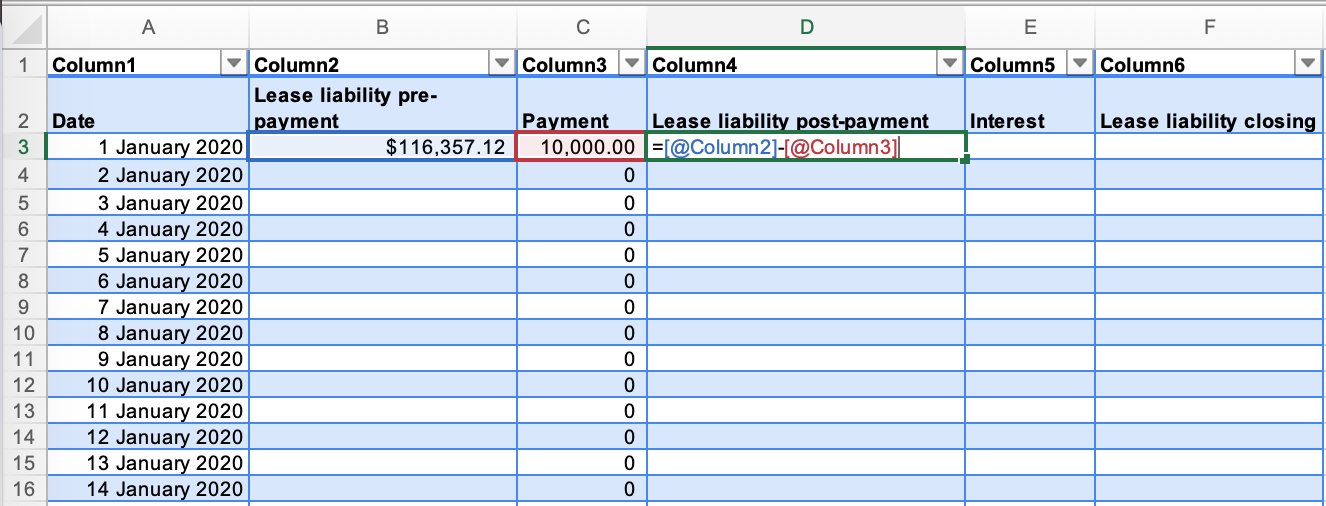• This is the lease liability's value post-payment. In this case, the lease liability pre-payment is \$116,357 less a \$10,000 payment resulting in \$106,357 lease liability balance post-payment.

### Step 5 - Calculate the daily interest rate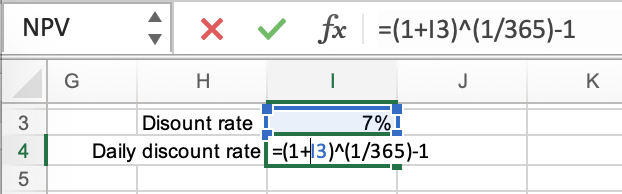• Given this is a daily calculation, it's necessary to convert the annual discount rate to a daily discount rate.

### Step 6 - Calculate the interest on the lease liability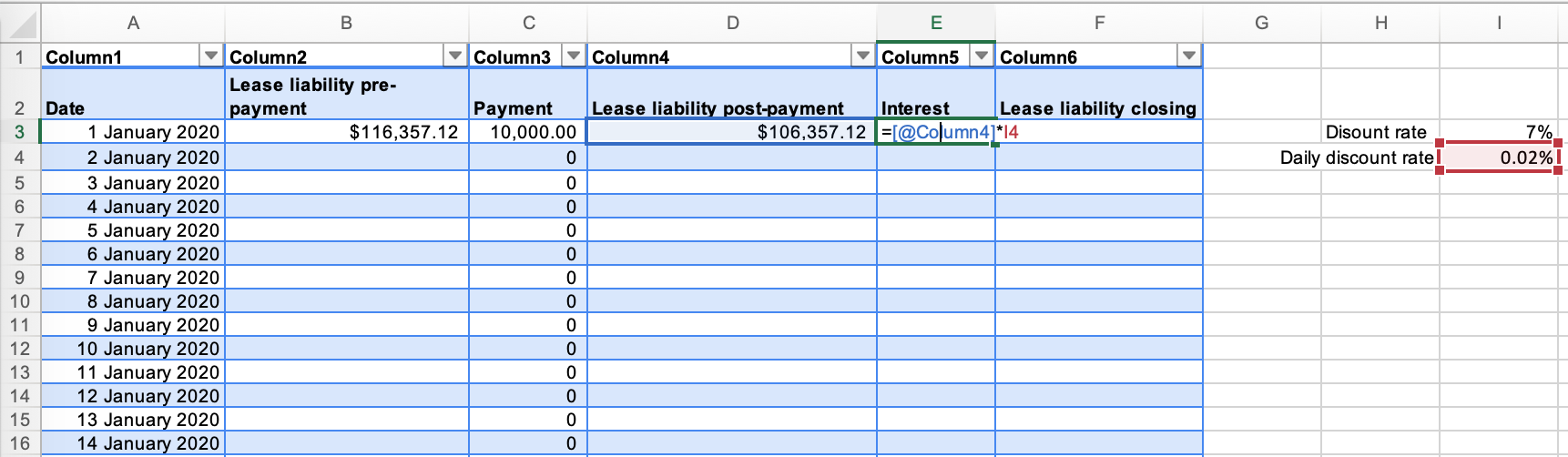• Apply the daily discount rate to the post-payment lease liability balance to calculate the interest incurred for the day.
• This amount will then be added to the lease liability value.

### Step 7 - Calculate the closing balance of the lease liability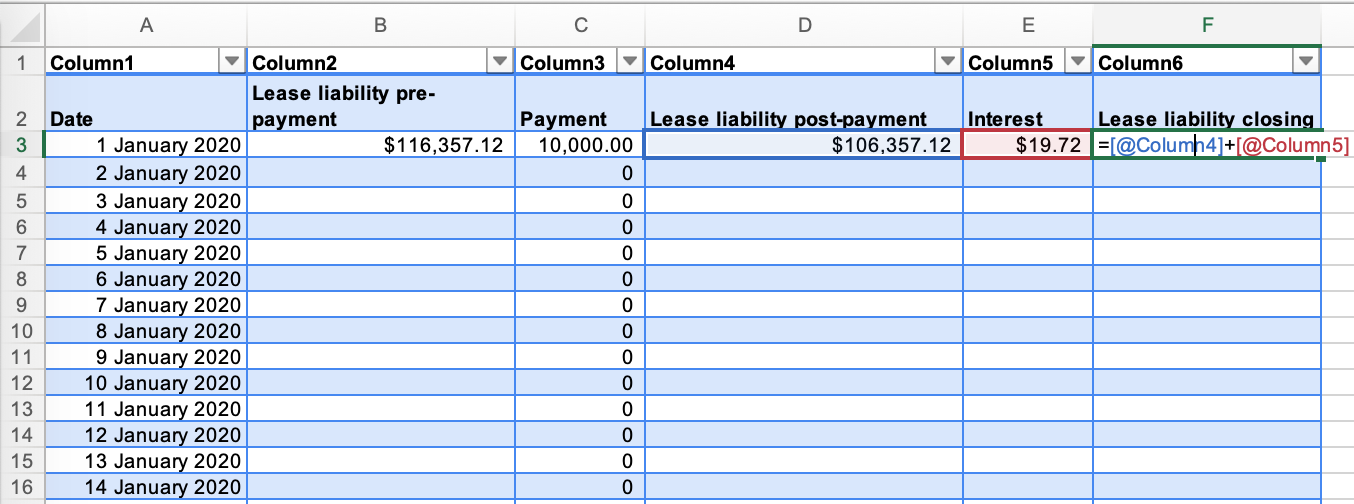• This is the closing balance for each applicable day.
• This balance will be brought forward as the opening balance for the next row in the Excel worksheet.

### Step 8 - Apply the formula in each cell to every row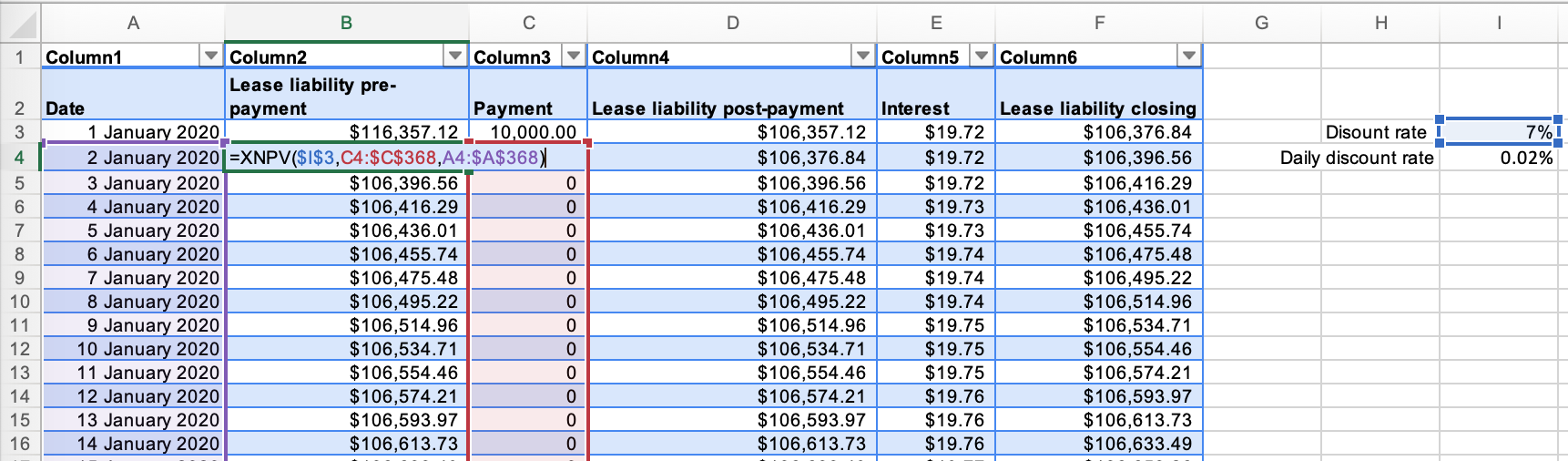• Ensure your formulas are picking up the correct cells.
• If they are not, that's probably the reason why the lease liability amortization schedule is not unwinding to zero.

### Step 9 - Ensure the lease liability unwinds to \$0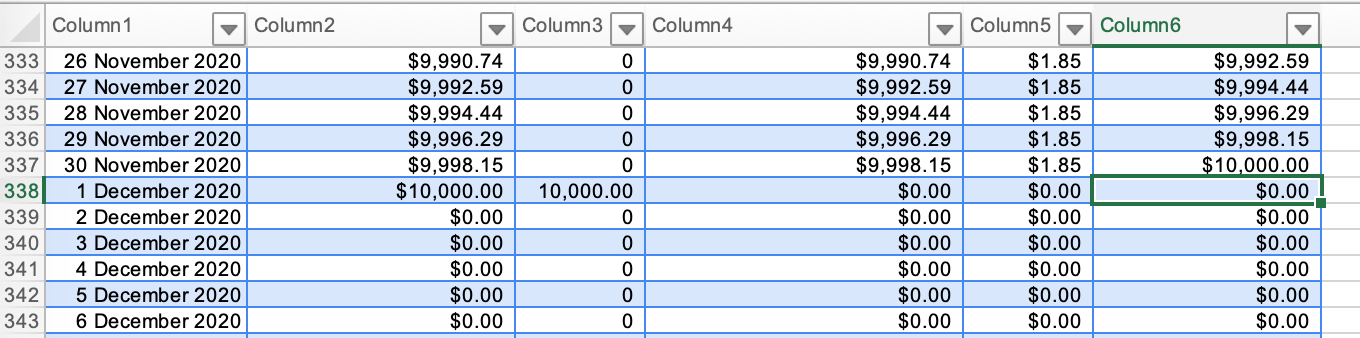• The best check to ensure you have calculated the lease liability amortization schedule is that the lease liability goes to zero!

# Conclusion

A daily lease amortization schedule is the most robust lease liability calculation available. As you can see, there are significantly more data points, but those data points give you flexibility when modification accounting occurs. For example, you know exactly what the closing balance is on March 25 as on March 26, the lessor communicated an increase in payments starting from April 1. As per the lease accounting standard, a remeasurement should occur on March 26 of the lease liability. Suppose you're using a monthly calculation, the only information available opening/closing balance of each month.

The lease liability amortization schedule is one of many pieces in the lease accounting puzzle. Here are some additional articles you might find helpful in broadening your understanding of lease accounting:

## FooterHere at Cradle, our mission is simple; it's at the foundation of everything that we do. We want to make accountants' lives easier by leveraging technology to free up their time to focus on running the business.

140 Yonge St.
Suite 200
Toronto, ON M5C 1X6

US
Suite #73591
Torrance, CA 90503
USA

### Legal